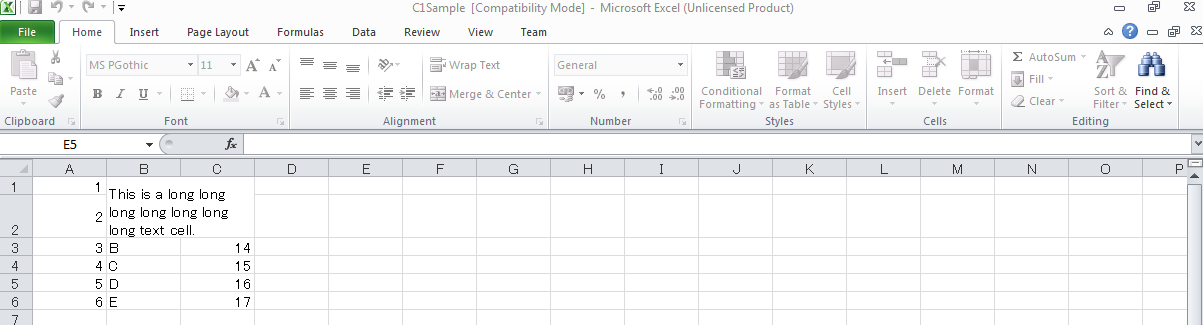﻿ Handling merged cell in C1Excel# Handling merged cell in C1Excel

C1Excel lets you merge multiple cells, so as to display them as a single cell. Further it even lets you wrap the cell text in case the text is too long. This blog discusses how you can enhance a cell which is a combination of the above two features. Suppose we have a merged cell, with multiple lines of wrapped text. By default C1Excel does not expands the row's height to display the complete content of the merged cell. Here we discuss how we can auto adjust the row height of such a merged cell in C1Excel to display the complete content. The final output in the Excel file would look like:Here is the logic integrated with C1Excel to achieve the above output:

• Check the size of the string in the merged cell.
• Check the size of the string which can be currently accommodated by merged cell area depending on its heigt and width.
• Add the difference between the above two sizes as the row height of the last row of merged cells.

This would increase the height of the merged cell and would display the complete text. Here is the code for the same:

``````

using (Graphics g = Graphics.FromHwnd(IntPtr.Zero))
{
foreach (XLCellRange cr in sheet.MergedCells)
{
// get value (unformatted at this point)
var value = cr.Value;
if (value == null || value.ToString().Length == 0) continue; // sanity value
var text = value.ToString();

// calculation merged cell size
int width = 0, height = 0, lastHeight = sheet.DefaultRowHeight;

for (int i = cr.ColumnFrom; i <= cr.ColumnTo; i++)       {         width += C1XLBook.TwipsToPixels(sheet.Columns[i].Width > 0 ? sheet.Columns[i].Width : sheet.DefaultColumnWidth);
}

for (int i = cr.RowFrom; i <= cr.RowTo; i++)       {         lastHeight = sheet.Rows[i].Height > 0 ? sheet.Rows[i].Height : sheet.DefaultRowHeight;
height += C1XLBook.TwipsToPixels(lastHeight);
}

// format value if cell has a style with format set
var s = sheet.MergedCells.Style;
if (s != null && s.Format.Length > 0 && value is IFormattable)
{
string fmt = XLStyle.FormatXLToDotNet(s.Format);
text = ((IFormattable)value).ToString(fmt, CultureInfo.CurrentCulture);
}

// get font (default or style)
var font = (s != null && s.Font != null) ? s.Font : book.DefaultFont;

// measuring text
var sz = Size.Ceiling(g.MeasureString(text + (s.WordWrap ? Environment.NewLine : string.Empty) + "XX", font, width));

// correct last height
var delta = C1XLBook.PixelsToTwips(sz.Height - height);
if (lastHeight + delta >= 0)
{
sheet.Rows[cr.RowTo].Height = lastHeight + delta;
}
}
}

``````GrapeCity

GrapeCity Developer Tools